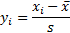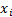/

### Function Description

Returns a standardised Quantile-Quantile (‘QQ’) plot with some extra comparisons. More precisely, returns a string which is the (temporary) code for a SmartChart that plots such information.

A standardised QQ-plot for a series of observations produces a plot of the observed quantile value versus the expected quantile value, with the series ‘standardised’ to have zero mean and unit standard deviation using a formula along the lines of:whereis the (sample) standard deviation of the data series.

The extra comparisons available are:

(a)    The corresponding Cornish Fisher (4th moment) asymptotic expansion, which in effect makes use of just the skew and kurtosis to estimate the shape of the QQ plot (set IncludeCF4 as true if you want these comparisons); and

(b)   The corresponding standard weighted cubic curve fit (set IncludeWCCF as true if you want these comparisons).

To allow users to plot several different series together, this function takes as input two different arrays, one containing all of the input data for all of the series (i.e. InputData), positioned consecutively and the other containing the number of entries in each series (i.e. InputDataSizes). So if InputDataSizes is {3,4} then two series are being analysed simultaneously, the first with three data points and the second with four, so InputData should have seven entries, the first three of which are for the first series and the last four for the second series.

For further details on how to use the ChartFormat parameter to format a chart see using the ChartFormat parameter.

Contents | Prev | Next